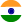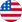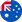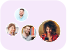24*7 support on WhatsAppChat NowProduct Edition:2003rd Edition
Author: Ch L. Hemleben, Laszlo Lovasz, Jozsef Pelikan
Book Name: Discrete Mathematics: Elementary and Beyond

# Discrete Mathematics: Elementary and Beyond 2003rd Edition Solutions

0 out of 5.0
51 reviews27 Students
have requested for homework help from this book

IntroductionDiscrete mathematics is quickly becoming one of the most important areas of mathematical research with applications to cryptography linear programming coding theory and the theory of computing. This book is aimed at undergraduate mathematics and computer science students interested in developing a feeling for what mathematics is all about where mathematics can be helpful and what kinds of questions mathematicians work on. The authors discuss a number of selected results and methods of discrete mathematics mostly from the areas of combinatorics and graph theory with a little number theory probability and combinatorial geometry. Wherever possible the authors use proofs and problem solving to help students understand the solutions to problems. In addition there are numerous examples figures and exercises spread throughout the book.L�szl� Lov�sz is a Senior Researcher in the Theory Group at Microsoft Corporation. He is a recipient of the 1999 Wolf Prize and the G�del Prize for the top paper in Computer Science. J�zsef Pelik�n is Professor of Mathematics in the Department of Algebra and Number Theory at E�tv�s Lor�nd University Hungary. In 2002 he was elected Chairman of the Advisory Board of the International Mathematical Olympiad. Katalin Vesztergombi is Senior Lecturer in the Department of Mathematics at the University of Washington.Read more

5
26
4
10
3
9
2
13
1
0

0

## Students who viewed this book also checked out